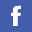BSc (Hons) in Mathematics and Statistics

Aims and Objectives

The B.Sc. programme is designed to provide students with an education that prepares them for careers as practicing mathematicians and/or statisticians. In order to accomplish this aim in the Hong Kong context, certain objectives have been incorporated into the curriculum as follows:

1. to give students a solid foundation in fundamental mathematics and statistics upon which to build their understanding of mathematical applications;
2. to provide a broad-based applied mathematics and statistics education;
3. to integrate computing literacy throughout students' studies because computers are indispensable tools for solving mathematical and statistical problems;
4. to foster in students an eagerness to solve scientific, industrial and commercial problems of concern to the local community and beyond, and
5. to teach students how to formulate suitable mathematical and statistical models for solving these problems.

Common Year 1 Science Core Courses:

BIOL1005 Introduction to Biology
CHEM1005 Introduction to Chemistry
COMP1005 Essence of Computing
MATH1025 Introduction to Mathematics and Statistics
PHYS1005 Introduction to Green Energy
SCIE1005 Integrated Science Laboratory

After completing the first year, students in Science Faculty may select BSc in Mathematics and Statistics as their major if they receive
(a) cGPA 2.0; and
(b) Grade C in MATH1025 Introduction to Mathematics and Statistics

At most one of BIOL1005, CHEM1005 and PHYS1005 can be replaced by a 3-unit Major elective course.

Core Courses (3 units each):
MATH1005 Calculus I
MATH2225 Calculus II
MATH2205 Multivariate Calculus
MATH2206 Probability & Statistics
MATH2207 Linear Algebra
MATH2215 Mathematical Analysis
MATH2216 Statistical Methods and Theory
MATH3205 Linear Programming and Integer Programming
MATH3206 Numerical Methods I
MATH3405 Ordinary Differential Equations
MATH3805 Regression Analysis
MATH3806 Multivariate Statistical Methods
MATH4998 Mathematical Science Project IHKBU/SFU Double Degree

The double degree approach leverages the quantitative and market skills of mathematics, statistics and actuarial science with corporate financial management practices.Department collaborates with overseas universities to offer joint/dual RPg programmes.

DetailsCourses

Major Discipline courses and free elective courses provided by the Department.

Details